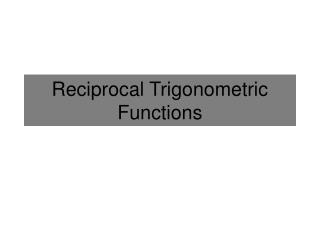# Reciprocal Trigonometric Functions - PowerPoint PPT PresentationDownload PresentationReciprocal Trigonometric Functions

Reciprocal Trigonometric FunctionsDownload Presentation## Reciprocal Trigonometric Functions

- - - - - - - - - - - - - - - - - - - - - - - - - - - E N D - - - - - - - - - - - - - - - - - - - - - - - - - - -
##### Presentation Transcript

1. Reciprocal Trigonometric Functions

2. Reciprocal The Reciprocal of any number x is: Ex: Find the reciprocal of 0.5x2 Because: Equivalent forms of this definition: The inverse of x is x-1. OR x and y are inverses if xy=1 Because:

3. Cosecant The cosecant function is the reciprocal of sine. It is defined as the following: Ex: Find the exact answer of csc(45°) CSC is the Abbreviation for cosecant There is no cosecant button on the calculator. You must use the definition above.

4. The Sine Function Graph In order to investigate the cosecant function, first examine the sine graph. Now find and graph all of the reciprocal values of sine.

5. The Cosecant Function Graph Find the reciprocal of the sine values. The errors are asymptotes. Plot the points.

6. The Cosecant Function Graph Domain: Range: Asymptotes All Reals except multiples of Pi. X = all multiples of Pi.

7. Secant The secant function is the reciprocal of cosine. It is defined as the following: Ex: Evaluate sec(72°) SEC is the Abbreviation for secant There is no secant button on the calculator. You must use the definition above.

8. The Cosine Function Graph In order to investigate the secant function, first examine the cosine graph. Now find and graph all of the reciprocal values of cosine.

9. The Secant Function Graph Find the reciprocal of the cosine values. The errors are asymptotes. Plot the points.

10. The Secant Function Graph Domain: Range: Asymptotes All Reals except

11. Cotangent The cotangent function is the reciprocal of tangent. It is defined as the following: Ex: Evaluate cot(0.5π) COT is the Abbreviation for secant The best form to use. There is no secant button on the calculator. You must use the definition above.

12. The Tangent Function Graph In order to investigate the cotangent function, first examine the tangent graph and all the values of cosine and sine. Remember, cotangent is cosine divided by sine. Now find and graph all of the values of cosine÷sine.

13. The Cotangent Function Graph Find the valuesof cosine divided by sine. The errors are asymptotes. Plot the points.

14. The Cotangent Function Graph Domain: Range: Asymptotes All Reals except multiples of Pi. All Reals. X = all multiples of Pi.